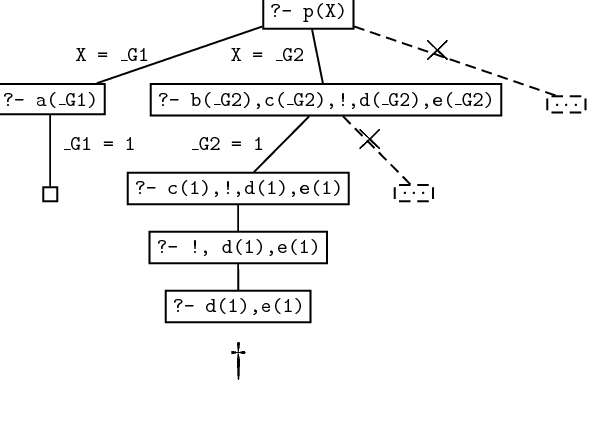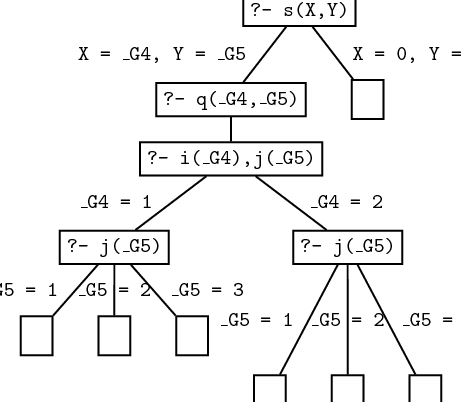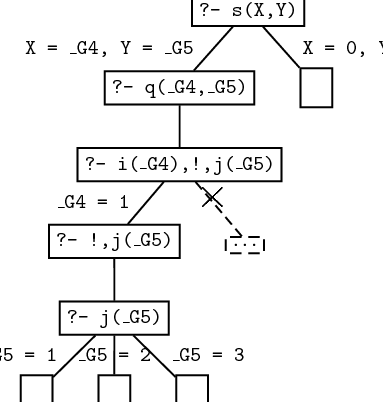### 10.1 The Cut

Automatic backtracking is one of the most characteristic features of Prolog. But backtracking can lead to inefficiency. Sometimes Prolog can waste time exploring possibilities that lead nowhere. It would be pleasant to have some control over this aspect of its behaviour, but so far we have only seen two (rather crude) ways of doing this: changing rule order, and changing goal order. But there is another way. There is a built-in Prolog predicate ! (the exclamation mark), called cut, which offers a more direct way of exercising control over the way Prolog looks for solutions.

What exactly is cut, and what does it do? It’s simply a special atom that we can use when writing clauses. For example,

p(X):-  b(X),  c(X),  !,  d(X),  e(X).

is a perfectly good Prolog rule. As for what cut does, first of all, it is a goal that always succeeds. Second, and more importantly, it has a side effect. Suppose that some goal makes use of this clause (we call this goal the parent goal). Then the cut commits Prolog to any choices that were made since the parent goal was unified with the left hand side of the rule (including, importantly, the choice of using that particular clause). Let’s look at an example to see what this means.

First consider the following piece of cut-free code:

p(X):-  a(X).

p(X):-  b(X),  c(X),  d(X),  e(X).

p(X):-  f(X).

a(1).    b(1).      c(1).      d(2).    e(2).    f(3).
b(2).      c(2).

If we pose the query p(X) we will get the following responses:

X  =  1  ;

X  =  2  ;

X  =  3  ;
no

Here is the search tree that explains how Prolog finds these three solutions. Note that it has to backtrack once, namely when it enters the second clause for p/1 and decides to unify the first goal with b(1) instead of b(2) .But now suppose we insert a cut in the second clause:

p(X):-  b(X),  c(X),  !,  d(X),  e(X).

If we now pose the query p(X) we will get the following responses:

X  =  1  ;
no

What’s going on here? Let’s consider.

1. p(X) is first unified with the first rule, so we get a new goal a(X) . By instantiating X to 1 , Prolog unifies a(X) with the fact a(1) and we have found a solution. So far, this is exactly what happened in the first version of the program.
2. We then go on and look for a second solution. p(X) is unified with the second rule, so we get the new goals b(X),c(X),!,d(X),e(X) . By instantiating X to 1 , Prolog unifies b(X) with the fact b(1) , so we now have the goals c(1),!,d(1),e(1) . But c(1) is in the database so this simplifies to !,d(1),e(1) .
3. Now for the big change. The ! goal succeeds (as it always does) and commits us to the choices made so far. In particular, we are committed to having X  =  1 , and we are also committed to using the second rule.
4. But d(1) fails. And there’s no way we can re-satisfy the goal p(X) . Sure, if we were allowed to try the value X=2 we could use the second rule to generate a solution (that’s what happened in the original version of the program). But we can’t do this: the cut has removed this possibility from the search tree. And sure, if we were allowed to try the third rule, we could generate the solution X=3 . But we can’t do this: once again, the cut has removed this possibility from the search tree.

If you look at the search tree, you’ll see that this all boils down to the following: search stops when the goal d(1) doesn’t lead to any node where an alternative choice is available. The crosses in the search tree indicate the branches that the cut trimmed away.One point is worth emphasising: the cut only commits us to choices made since the parent goal was unified with the left hand side of the clause containing the cut. For example, in a rule of the form

q:-  p1,...,pn,  !,  r1,...,rm

when we reach the cut it commits us to using this particular clause for q and it commits us to the choices made when evaluating p1,...,pn . However, we are free to backtrack among the r1,...,rm and we are also free to backtrack among alternatives for choices that were made before reaching the goal q . A concrete example will make this clear.

First consider the following cut-free program:

s(X,Y):-  q(X,Y).
s(0,0).

q(X,Y):-  i(X),  j(Y).

i(1).
i(2).

j(1).
j(2).
j(3).

Here’s how it behaves:

?-  s(X,Y).

X  =  1
Y  =  1  ;

X  =  1
Y  =  2  ;

X  =  1
Y  =  3  ;

X  =  2
Y  =  1  ;

X  =  2
Y  =  2  ;

X  =  2
Y  =  3  ;

X  =  0
Y  =  0;
no

And this is the corresponding search tree:Suppose we add a cut to the clause defining q/2 :

q(X,Y):-  i(X),  !,  j(Y).

Now the program behaves as follows:

?-  s(X,Y).

X  =  1
Y  =  1  ;

X  =  1
Y  =  2  ;

X  =  1
Y  =  3  ;

X  =  0
Y  =  0;
no

Let’s see why.

1. s(X,Y) is first unified with the first rule, which gives us a new goal q(X,Y) .
2. q(X,Y) is then unified with the third rule, so we get the new goals i(X),!,j(Y) . By instantiating X to 1 , Prolog unifies i(X) with the fact i(1) . This leaves us with the goal !,j(Y) . The cut, of course, succeeds, and commits us to the choices made so far.
3. But what are these choices? These: that X  =  1 , and that we are using this clause. But note: we have not yet chosen a value for Y .
4. Prolog then goes on, and by instantiating Y to 1 , Prolog unifies j(Y) with the fact j(1) . So we have found a solution.
5. But we can find more. Prolog is free to try another value for Y . So it backtracks and sets Y to 2 , thus finding a second solution. And in fact it can find another solution: on backtracking again, it sets Y to 3 , thus finding a third solution.
6. But those are all alternatives for j(X) . Backtracking to the left of the cut is not allowed, so it can’t reset X to 2 , so it won’t find the next three solutions that the cut-free program found. Backtracking over goals that were reached before q(X,Y) is allowed however, so that Prolog will find the second clause for s/2 .

Here’s the corresponding search tree:© 2006-2012 Patrick Blackburn, Johan Bos, Kristina Striegnitz